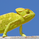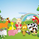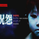1883 vizualizações
Perform higher order differencing through convolution, the result is equivalent to cascading N momentum oscillators of periods P:

`mom(mom(mom(mom(x,P)...,P)`

Settings

length - Period of the oscillator, indicate the lag to use (equivalent to the period in a momentum oscillator)

order - Differencing order, indicate how many times differencing is performed (number of times a momentum oscillator is cascaded)

src - Input of the indicator

Usage

Differencing consists in subtracting an input to a previous input, this is what the momentum oscillator performs. This is often done in order to remove longer-term variations in the price. Differencing also induces a 90-degree phase shift for all sinusoids in a signal, this is why oscillators can have this leading effect, as such higher differencing can sometimes help have a faster and more visible lead.

In red the indicator with period 50 and differencing order 2, below a momentum oscillator of the same period.

It is important to note that differencing is an operation that increases noise, in fact, you might have seen some oscillators use the median price hl2 instead of the closing price, this is because the median price contains less noise than the closing price, as such more differencing require a smoother input.

Here both the sma and the oscillator period are equal to 20 with a differencing order of 5.

In time series analysis the order of differencing is chosen depending on the order of integration, more simply put we should choose a differencing order that responds to the question: "How many time should I differentiate my time series so that the result is stationary?", for stocks prices this differencing order should be 1.

Technically speaking differencing orders higher than 3 might be overkill, as higher orders return noisier outputs that might no longer be representative of the original input.

here a period of 14 with differencing order of 20 is used, we can see more periodic results but they are not really representative of the closing prices.

Details

Simple differencing is actually achieved thought convolution, if we take a first-order difference x - x(1), we can see that this is equivalent to 1*x + -1*x(1), the coefficients are 1 and -1, for the momentum oscillator the difference is that the coefficients include 0 values. So we only need a function generating the coefficients of our Nth order difference oscillator, in order to get them lets analyze the impulse response of a cascaded change function.

Here 5 change function are cascaded, the coefficients are: (1,-5,10,-10,5,-1)

If you look at these coefficients and the ones of higher/lower order differences we can deduce various things

• The impulse response is symmetric
• The first coefficient is always 1 and the last always -1
• The number of coefficients increase with higher differencing orders
• The sign of the current coefficient is different from the sign of the previous one

From the shape of the impulse response, we can deduce that the coefficients of an Nth order differencing operator is a windowed series of 1,-1,1...,-1, and that's actually the case, for an Nth order differencing operator the values of this window are given by the Nth row of the Pascal triangle.

There are various ways to get the values in the row of the Pascal triangle, one involving using the combination formula, however, we can do it way faster by using the recursive formula used in line number 13. Now that we have our coefficients we only need to separate them with 0 values and that's all.

Conclusion

We can see that oscillators are noisier than the original input signal, this is can be a desired effect in order to make lagging indicators more reactive, but it can also be overlooked due to the results appearing leading the price or just looking more predictable, however, we should note that higher-order differencing does not provide a consistent nor reliable solution toward minimizing lag, nor does classical oscillators.

The indicator is not useful, but if for some reason you require a lot of differencing operations to be done and don't want to use consecutive change or mom functions, then this script might results useful to you.

Script de código aberto

No verdadeiro espírito TradingView, o autor deste script o publicou com código aberto, para que os traders possam compreendê-lo e verificá-lo. Um brinde ao autor! Você pode usá-lo gratuitamente, mas a reutilização deste código em uma publicação é regida pelas Regras da Casa. Você pode favoritá-lo para utilizá-lo em um gráfico.

Quer usar esse script no gráfico?
Patreon: https://www.patreon.com/alexgrover

You can also check out some of the indicators I made for luxalgo : https://www.tradingview.com/u/LuxAlgo/#published-scripts

## ComentáriosGreat explanation and a great oscillator.
Together with a second filter as source the indicator shows really good results.
RespostaHi Alex, as others traders comments below I think this could be a nice idea for develop further stronger predictive indicators in future. I also agreed as Alex you mentioned in the conclusion section "this indicator is not useful" I would said is could be useful if combined with others indicators, but if use it just standalone I do agree that this indicator is not that useful too.

Appreciated your effort and shared Alex; wish you have a beautiful weekend <3
Respostano，its very useful,but you dont know how to use it ,as many of your indictor
Resposta